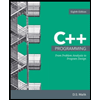Question

Consider the Sort-and-Count algorithm explained in section 5.3 of our text: "Counting Inversions"

Suppose that the initial list is:

92   71   36   91   27   48   14   34   81   26   24   65   78   51   37   22

Sort-and-Count makes two recursive calls. The first recursive call inputs the first half of the initial list:

92   71   36   91   27   48   14   34

and returns the sorted version of the first half, as well as the number of inversions found in the first half (22). The second recursive call inputs the second half of the initial list:

81   26   24   65   78   51   37   22

and returns the sorted version of the second half, as well as the number of inversions found in the second half (19). Sort-and-Count then calls Merge-and-Count. To Merge-and-Count, Sort-and-Count passes the sorted versions of the two halves of the original list:

14   27   34   36   48   71   91   92, and

22   24   26   37   51   65   78   81

Merge-and-Count begins merging the two half-lists together, while counting inversions.

14   27   34   36   48   71   91   92      22   24   26   37   51   65   78   81

14   22   24   26   27   34   36   37   48   51   65   71   78   81   91   92

7    7    7                   4         3    3         2    2     (inversions)

The inversions counted during the merge are shown in the last line above. The total number of inversions counted between the two sorted halves is

7+7+7+4+3+3+2+2 = 35.

Therefore the total number of inversions in the original list is

22+19+35 = 76.

Do the same work as above, except starting with this initial list:

26   81   87   32   66   72   86   97   23   48   14   71   89   18   49   62

Expert Solution

### Want to see the full answer?

Check out a sample Q&A hereStudents who’ve seen this question also like:C++ Programming: From Problem Analysis to Program Design
8th Edition
ISBN: 9781337102087
Author: D. S. Malik
Publisher: Cengage Learning